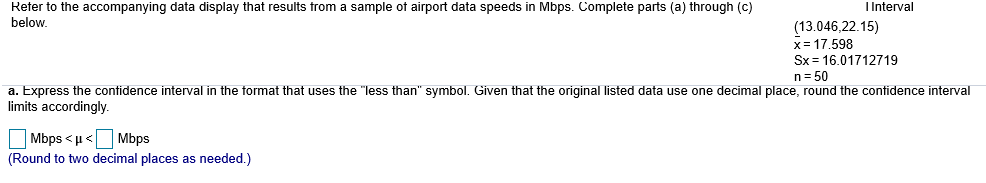# Reter to the accompanying data display that results trom a sample ot airport data speeds in Mbps. Complete parts (a) through (c)below.I Interval13.046,22.15)x-17.598Sx 16.01712719n 50a. Express the contidence interval in the tormat that uses the "less than" symbol. Given that the original listed data use one decimal place, round the contidence intervalimits accordingly.(Round to two decimal places as needed.)

Questionhelp_outlineImage TranscriptioncloseReter to the accompanying data display that results trom a sample ot airport data speeds in Mbps. Complete parts (a) through (c) below. I Interval 13.046,22.15) x-17.598 Sx 16.01712719 n 50 a. Express the contidence interval in the tormat that uses the "less than" symbol. Given that the original listed data use one decimal place, round the contidence interval imits accordingly. (Round to two decimal places as needed.) fullscreen
check_circleExpert Solution
Step 1

Confidence Interval:

The form of the confidence interval is,

CI = Point estimate ± Margin of error.

α is the level of significance.

The approximate 100*(1–α)% confidence interval for the population mean will be obtained using the formula given below:

Step 2

Express the confidence interval in the format that uses “less than” symbol:

Denote the population mean speed of airport data as μ.

The data represents the confidence interval for the population mean speed of airport data.

The...

### Want to see the full answer?

See Solution

#### Want to see this answer and more?

Solutions are written by subject experts who are available 24/7. Questions are typically answered within 1 hour*

See Solution
*Response times may vary by subject and question
Tagged in

### Other